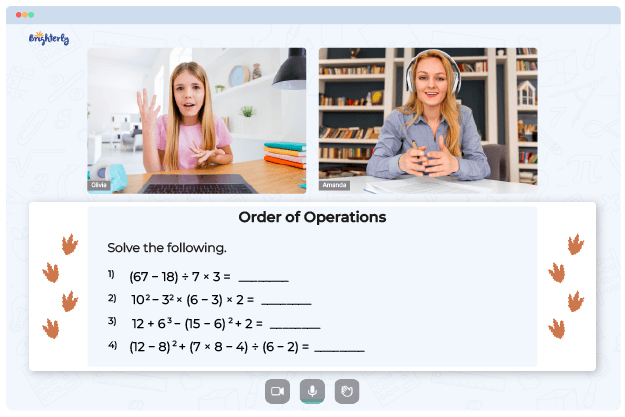# Order of Operations with Integers Worksheet

The order of operations is a set of rules that dictate the sequence in which mathematical operations should be performed when solving an expression. The order of operations with integers worksheet will help students to learn and reinforce their knowledge of the concept of orders of operations with integers.

## How Does Order of Operations with Integers Worksheet Work?

Our operations with integers worksheet includes a series of mathematical expressions involving integers where students are required to follow the PEMDAS rule to evaluate each expression correctly. The acronym PEMDAS (Parentheses, Exponents, Multiplication and Division, Addition and Subtraction) is often used as a mnemonic to remember the order of operations.

Math for Kids

Is Your Child Struggling With Math?
1:1 Online Math TutoringUsing the operations with integers worksheet pdf, students will be able to understand this concept better. Also, the integer order of operations worksheet includes word problems that require students to use the order of operations to solve real-life situations involving integers.### Order of Operations with Integers Worksheet PDF

Integer Operations Worksheet### Order of Operations with Integers Worksheet PDF

Order Of Operations With Integers Worksheet### Order of Operations with Integers Worksheet PDF

Operations With Integers Worksheet### Order of Operations with Integers Worksheet PDF

Operations With Integers Worksheet PDF

Need help with Order of Operations?• Does your child need additional assistance with mastering order of operations?
• Start learning with an online tutor.

Is your child finding it challenging to understand order of operations? An online tutor could be the solution.